# Consider the following reaction at equilibrium: NiO(s)+CO(g) 2 Ni(s) + CO2(g). Select all of the following...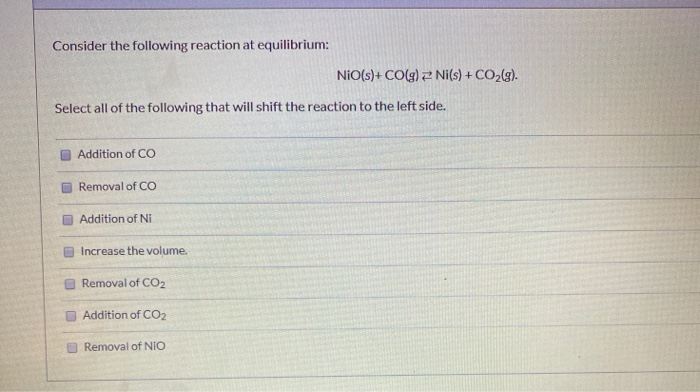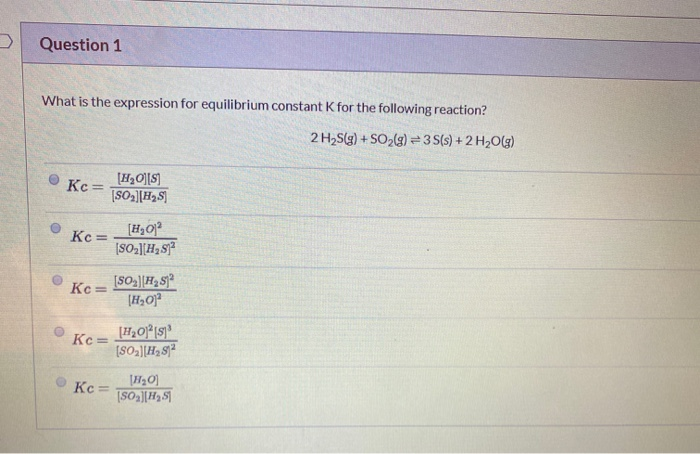Consider the following reaction at equilibrium: NiO(s)+CO(g) 2 Ni(s) + CO2(g). Select all of the following that will shift the reaction to the left side. Addition of CO Removal of CO Addition of Ni Increase the volume. Removal of CO2 Addition of CO2 Removal of NIO
Question 1 What is the expression for equilibrium constant for the following reaction? 2 H2S(g) + SO2(g) = 35(s) + 2 H2O(g) Kc= [HQ][S] [SO][H251 Kc= [HO] [H,872 Kc=  [H2 51 (H20 Kc= [H20] [51 (S02] [H282 Kc= [H0 [SO][251

--> kc = [CO2]/[CO]

Adding of CO will shift reaction towards right.

Removal of CO will shift reaction towards left.

Adding of Ni will have no effect.

Increase in volume will shift reaction towards more no. of gaseous molecules. So here, both side gaseous moles are equal hence no effect.

Removal of CO2 will shift reaction towards right.

Addition of CO2 will shift reaction towards left.

Removal of NiO will have no effect.

Option 2 and 6 will shift reaction towards left.

--> reaction is--

2H2S(g) + SO2(g) --> 3S(s) + 2H2O(g)

Kc = [H2O]2/[H2S]2[SO2]

Option 2.

If you have any query please comment

If you satisfied with the solution please rate it thanks...

##### Add Answer of: Consider the following reaction at equilibrium: NiO(s)+CO(g) 2 Ni(s) + CO2(g). Select all of the following...
Similar Homework Help Questions
• ### Consider the reaction: NiO(s)+CO(g)⇌Ni(s)+CO2(g) Kc=4000.0 at 1500 K When calculating the answer, do not round to...

Consider the reaction: NiO(s)+CO(g)⇌Ni(s)+CO2(g) Kc=4000.0 at 1500 K When calculating the answer, do not round to the appropriate number of significant figures until the last calculation step. Part A If a mixture of solid nickel(II) oxide and 0.11000 M carbon monoxide is allowed to come to equilibrium at 1500 K, what will be the equilibrium concentration of CO2?

• ### For which reaction will Kp = Kc? ONiCO3(s) = NiO(s) + CO2(g) O 2 HgO(s) =...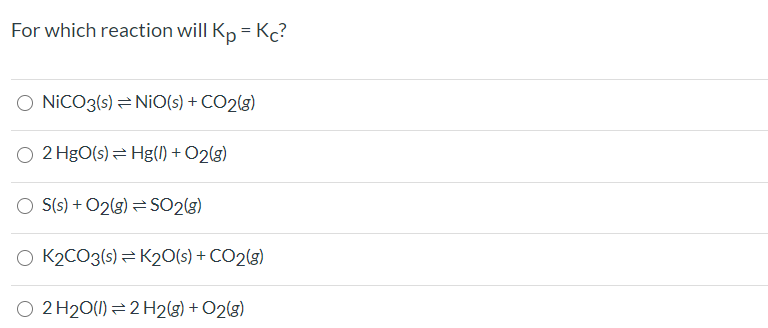For which reaction will Kp = Kc? ONiCO3(s) = NiO(s) + CO2(g) O 2 HgO(s) = Hg(1) + O2(8) OS(s) + O2(8) =SO2(g) K2CO3(s) =K20(s) + CO2(g) O 2 H2O(1) = 2 H2(g) + O2(g) Show transcribed image text

• ### 4. Consider the following reaction at equilibrium CO(g) + H2O(g) + CO2(g) + H2(g) 2.50 mole...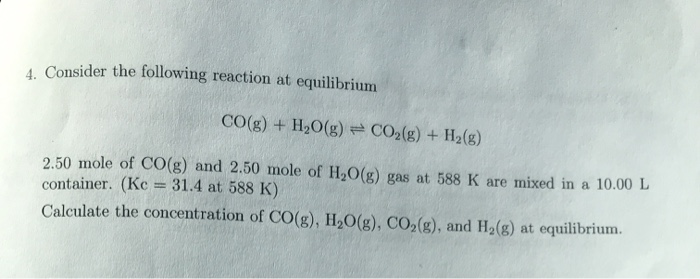4. Consider the following reaction at equilibrium CO(g) + H2O(g) + CO2(g) + H2(g) 2.50 mole of CO(g) and 2.50 mole of H2O(g) gas at 588 K are mixed in a 10.00 L container. (Kc = 31.4 at 588 K) Calculate the concentration of CO(g), H2O(g), CO (g), and H.(g) at equilibrium. 5. Consider the following reaction: CO(g) + H2O(g) + CO2(g) + H2(g) (a) If a 10.00L container has 2.50 mole of CO(g), 2.50 mole of H2O(g), 5.00 mole...

• ### Given the following balance CO (g) + H20 (g)s CO2 (g) + H2 (g) Kc 26...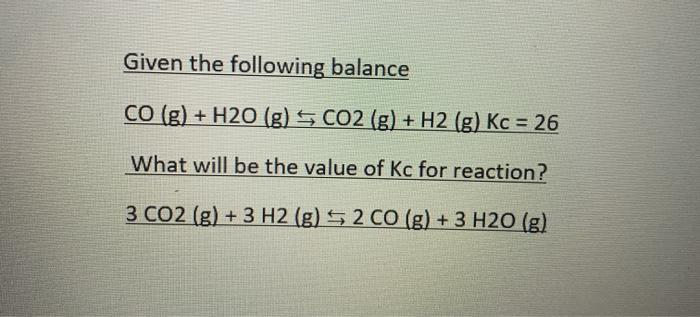Given the following balance CO (g) + H20 (g)s CO2 (g) + H2 (g) Kc 26 What will be the value of Kc for reaction? 2 CO (g) +3 H20 (g) 3 CO2 (g)+3 H2 (g)

• ### Nickel sulfite can be prepared by the following reaction: 2 Ni (s) + 1/4S8 (s) +...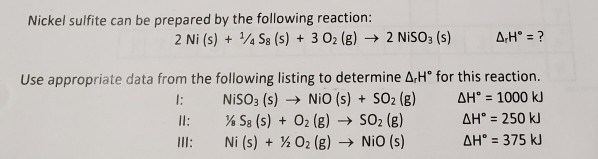Nickel sulfite can be prepared by the following reaction: 2 Ni (s) + 1/4S8 (s) + 3 02 (g) → 2 NISO: (s) A.Hº = ? Use appropriate data from the following listing to determine A,Hº for this reaction. 1: NISO: (s) → NIO (s) + SO2 (g) AH° = 1000 kJ ll: % S8 (s) + O2(g) → SO2 (g) AH = 250 kJ Ill: Ni (s) + % 02 (g) → NIO (s) AH = 375 kJ

• ### 27. Consider the following reaction and its equilibrium constant: SO2(g) + NO2(g) = SO3(g) + NO(g)...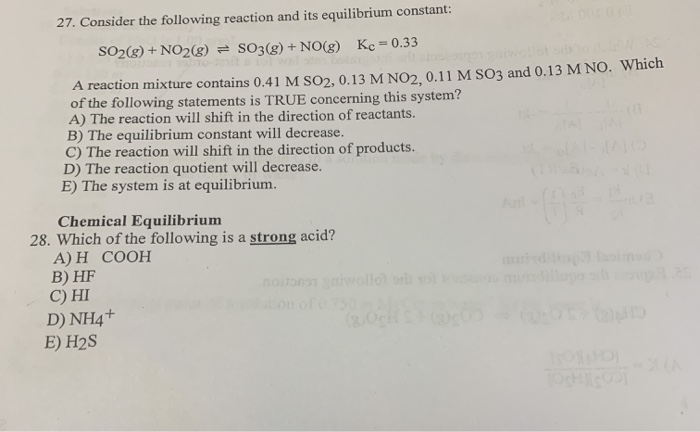27. Consider the following reaction and its equilibrium constant: SO2(g) + NO2(g) = SO3(g) + NO(g) Kc = 0.33 A reaction mixture contains 0.41 M SO2, 0.13 M NO2.0.11 M SO3 and 0.13 M NO. Which of the following statements is TRUE concerning this system? A) The reaction will shift in the direction of reactants. B) The equilibrium constant will decrease. C) The reaction will shift in the direction of products. D) The reaction quotient will decrease. E) The system...

• ### Q(18) NI(CO)49) - Ni(s) + 4 CO(g)Adding nickel (solid) to this reaction will cause the equilibrium...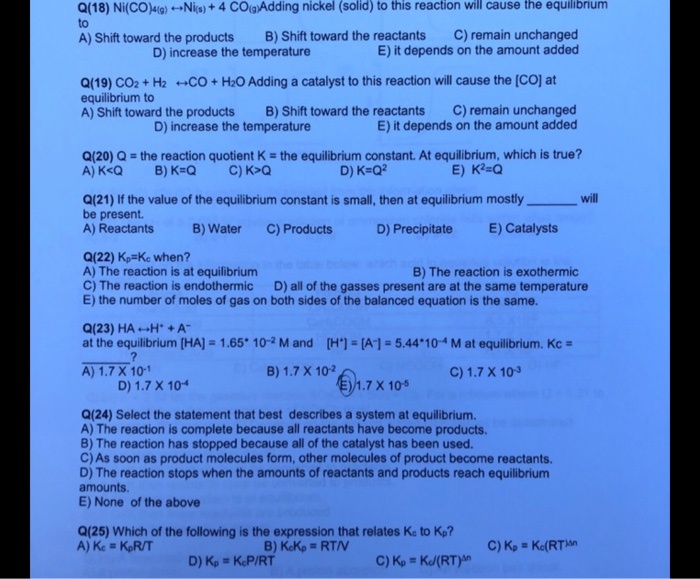Q(18) NI(CO)49) - Ni(s) + 4 CO(g)Adding nickel (solid) to this reaction will cause the equilibrium to A) Shift toward the products B) Shift toward the reactants C) remain unchanged D) increase the temperature E) it depends on the amount added Q(19) CO2 + H2 -CO + H2O Adding a catalyst to this reaction will cause the COD at equilibrium to A) Shift toward the products B) Shift toward the reactants C) remain unchanged D) increase the temperature E) it...

• ### 26) 26) Consider the following reaction and its equilibrium constant Ke 1.10 CO2(8)+ 4 Cu(s) +2 H20(g) 4 CuO(s)+ CH...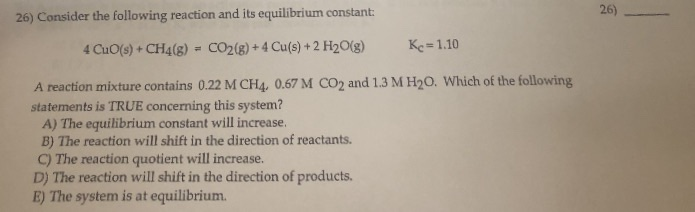26) 26) Consider the following reaction and its equilibrium constant Ke 1.10 CO2(8)+ 4 Cu(s) +2 H20(g) 4 CuO(s)+ CH4(g) A reaction mixture contains 0.22 M CH4, 0.67 M CO2 and 1.3 M H20. Which of the following statements is TRUE concerning this system? A) The equilibrium constant will increase. B) The reaction will shift in the direction of reactants C)The reaction quotient will increase. D) The reaction will shift in the direction of products. E) The system is at...

• ### The following endothermic reaction is at equilibrium. 2 CO(g) + O2(g) 42 CO2 (g) What is...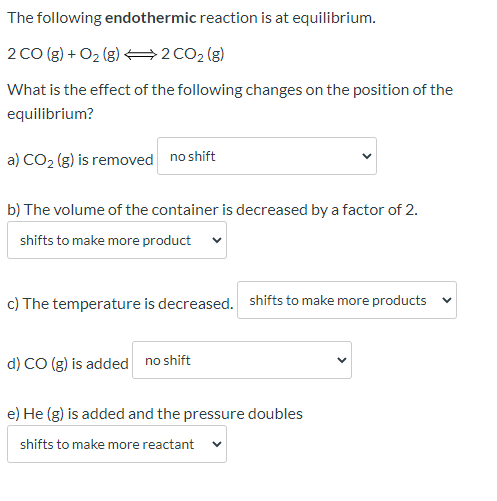The following endothermic reaction is at equilibrium. 2 CO(g) + O2(g) 42 CO2 (g) What is the effect of the following changes on the position of the equilibrium? a) CO2 (g) is removed no shift b) The volume of the container is decreased by a factor of 2. shifts to make more product c) The temperature is decreased. shifts to make more products d) CO (g) is added no shift e) He (g) is added and the pressure doubles shifts...

• ### Chemical Equilibrium Characteristics Consider the reaction: H20(g)Co(g) = H2(g) Co2(g) Which the following statements a...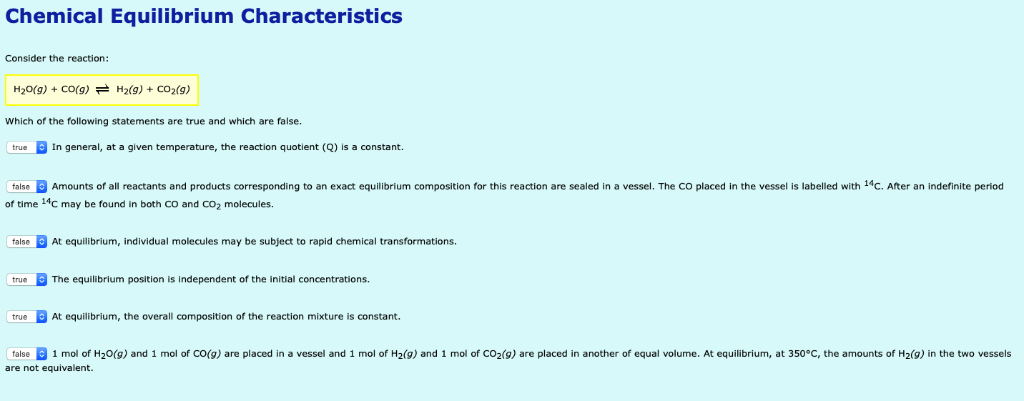Chemical Equilibrium Characteristics Consider the reaction: H20(g)Co(g) = H2(g) Co2(g) Which the following statements are true and which are false. In general, at a given temperature, the reaction quotient (Q) is a constant. true Amounts of all reactants and products corresponding to an exact equilibrium composition for this reaction are sealed in a vessel. The CO placed in the vessel is labelled with 14C. After an indefinite period false time 14C may be found in both CO and CO2 molecules....

Free Homework App